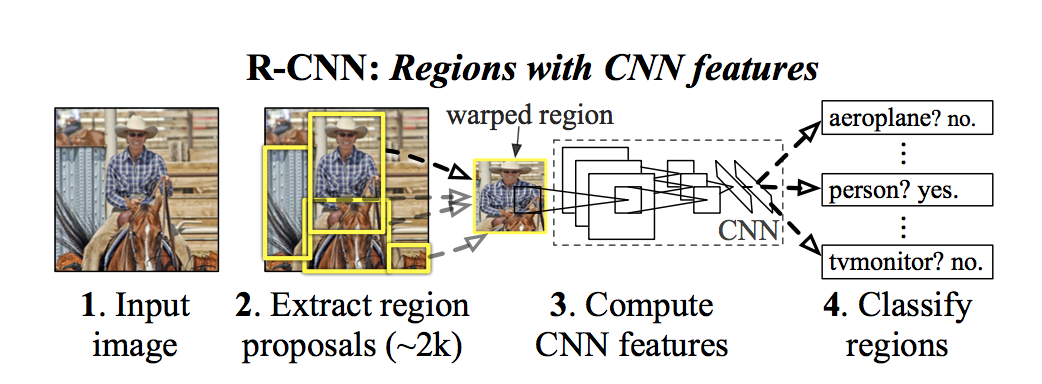R-CNN

Rich feature hierarchies for accurate object detection and semantic segmentation Tech report

Posted by GwanSiu on July 21, 2017

1. 目标检测的根本问题(Classification+Regression)

1. 分类问题: 对候选区域判断是否有物体存在
2. 回归问题: 对于有物体存在的区域进行bounding box regression, 即:预测出bounding box四个点的坐标。 目标检测要回答的问题：什么位置有什么物体？
目标检测主要思路: 候选区域选择—>对候选区域进行特征提取—>分类—>对选择好的候选区域进行回归

2. R-CNN 的主要贡献

1. 将CNN结构直接作用于候选区域上，实现提取特征,定位和分割的操作。CNN具有非常好的特征提取功能，但CNN对于输入的大小是固定的，候选区域(proposed region)在选择上是可大可小，具有多尺度特性，因此CNN不能直接作用在候选区域上。本文解决的办法很简单，将所有候选区域(proposed region) Wrap成统一大小。
2. 当训练样本较少时，先使用额外的训练样本进行预训练，接着使用训练样本微调(fine tune)网络，同样能达到较好的效果。

3. R-CNN的结构RCNN主要包括三个部分: Region Proposal, CNN for feature extracture and a set of specific linear CNN。最后将提取好的特征使用bounding box regression算法精准定位。

1. Region Proposal: Region Proposal部分是产生category-independent region proposals， 也定义了这个网络可以检测的物体的种类，这就是为什么背景中有些物体不会被检测出来，因为检测的物体是需要被定义的，告诉检测系统，需要检测哪些物体。 什么是category-independent? 候选区域内只有一个物体，不存在物体之间overlapping的现象，通俗讲，就是候选区域(region proposal)中是狗的，就不会出现一只猫和狗在一起的现象，并且每个region proposals之间相互独立，互不干扰。 文中列举了许多region proposal的方法，本文使用的是“selective search”的方法,fast mode.详情可见.
2. 特征提取: 将region proposal统一wrap成固定大小，减去均值后输入CNN网络中提取出4098维特征向量，最后用linear SVM分类。这里的Linear SVM是进行二元分类的，由于分类中负样本比较多，因此采取了“hard negative mining”.作者在文中提到，不同网络对RCNN最后效果影响是非常大的，作者说VGG会比Krizhevsky 12年提出的网络结构要好，显然是符合逻辑的，因为VGG够深，特征提取的效果更好。
3. 测试阶段: 将测试图片使用region proposal的方法产生2000个proposed region, wrap成统一大小输入到神经网络中提取特征，最后使用linear SVM进行分类。在对一张图片的所有regions评分后用greedy non-maximum suppression的方法对scored region进行筛选，去掉具有高重叠率的区域，让包含某一个物体的region proposal最好剩下一个，最后使用bounding box regression精准定位。
4. 为什么使用SVM而不是softmax?作者在文中附录有所提及，结论是softmax的效果会变得较差。通俗来讲，神经网络对正负样本的数量分布比较敏感，如果要fine tune整个network使其不过拟合, 需要比这大30倍的样本，但这个数据集很小，不满足要求。而SVM则恰恰相反，hard negative mining，在正负样本不平衡的情况下，依然可以学到而不会过拟合，原因是SVM分类的超平面主要是支持向量(support vector)贡献的，非支持向量(support vector)对分类超平面是没有贡献的。

4. 训练RCNN

RCNN需要训练三个部分: CNN特征提取模型(微调)，分类模型linear SVM 以及定位模型bounding box regeression。

1. CNN特征提取模型: 使用ILSVRC2012数据集进行预训练，再根据特定的数据集进行微调，将ILSVRC数据集中的物体类别N=200，改成N=10(特定数据集的类别)。使用SGD进行训练，与ground true的IoU大于0.5的标位正，其余的标为负。
2. Linear SVM: 将提取的特征输入到所有SVM中进行二元分类，训练模式是hard negative mining.对所有positive region 进行评分。
3. bounding box regression: 输入是提取的特征向量，输出bounding box坐标。
Bounding Box Regression
输入为$(P,G)$,其中$P=(P_{x},P_{y},P_{w},P_{h})$是region proposal的中心坐标以及宽和高。$G=(G_{x},G_{y},G_{w},G_{h})$是ground true的中心坐标以及宽和高。Bounding box regression就是找到四个函数$d_{x}(P),d_{y}(P),d_{w}(P),d_{h}(P)$,将P映射到G，其中前两个是尺度不变拉伸，后两个是log尺度拉伸。
$\begin{equation} \hat{G_{x}} = P_{w}d_{x}(P)+P_{x} \tag{1}\\ \hat{G_{y}} = P_{h}d_{y}(P)+P_{y} \\ \hat{G_{w}} = P_{w}exp(d_{w}(P)) \\ \hat{G_{h}} = P_{h}exp(d_{h}(P)) \\ \end{equation}$

$\min_{w_{\ast}}\sum_{i}^{N}(t_{\ast}^{i}-\hat{w}^{T}_{\ast}\phi_{5}(P))^{2}+\lambda||\hat{w}_{\ast}||^{2} \tag{2}$

$\begin{equation} t_{x}=(G_{x}-P_{x})/P_{w} \tag{3}\\ t_{y}=(G_{y}-P_{y})/P_{h}\\ t_{w}=\text{log}(G_{w}/P_{w})\\ t_{h}=\text{log}(G_{h}/P_{h})\\ \end{equation}$

5 总结

RCNN的优点:

1. 与overfeat相比(使用的sliding window方法提取region proposal， sliding window属于一种遍历方法，会产生大量无用的overlapping的候选区域)，Region proposal则很大程度减少候选区域的个数，从而缩短检测时间。
2. CNN具有强大的特征提取功能，但Region proposal尺度不一，为了二者相互兼容，使用了wrap操作。但这一定程度上造成了物体的形变，后续论文中提出使用SPPnet进行改进。
3. 使用微调(fine tune)，将现有网络直接应用于目标检测。

RCNN的缺点： 检测时间很长，原因在于，每次一张图片提取2000个region proposal输入到网络，region proposald 操作耗费了主要的时间。